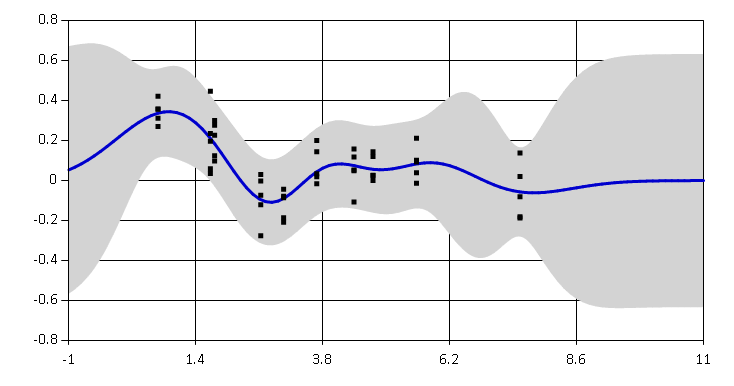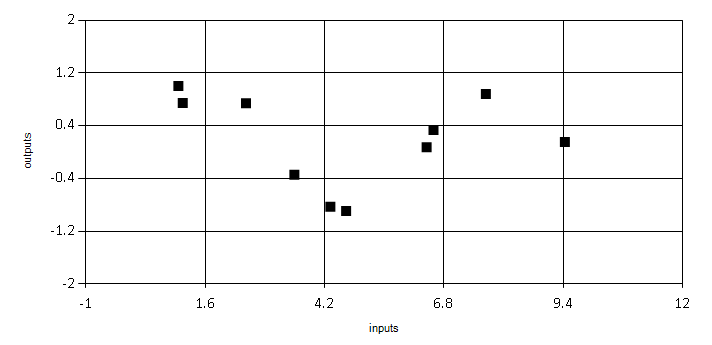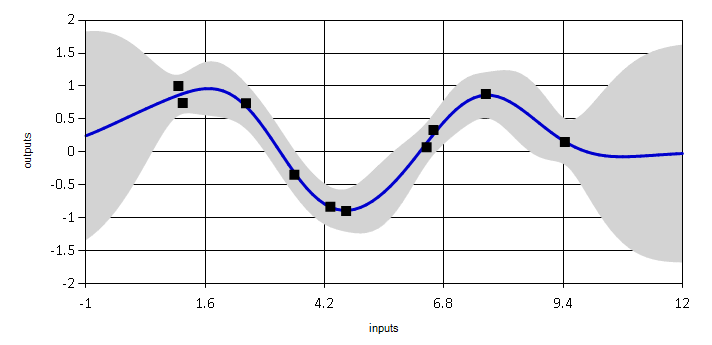# Ariadne: F# library for Gaussian process regression

Ariadne is an F# library for fitting Gaussian process regression models developed by Evelina Gabasova.## What are Gaussian processes?

Let's start with an example. Assume we have a set of noisy time series observations, like the one below. We would like to model relationship between the input values and the outputs.When using standard regression models, like linear regression, we have to assume there is a specific parametric relation between the observed data.

With Gaussian process regression, we have to use a different types of assumptions. In this case, we will assume that the underlying function is quite smooth with some amount of noise. What Gaussian processes give us is a flexible regression function which takes account of noise in observed data and provides a measure of uncertainty in the regressed values.

In the example below, the blue line represents the predicted mean value of the estimated function. The grey region shows the distance of +/- two standard deviations from the mean, which corresponds to 95% confidence interval. This quantity shows how uncertain the model is about value of the function. Note that the grey area is wider in locations where there are no observations, and shrinks when a value is observed.If you want to learn more about Gaussian processes, the best resource is the Gaussian Processes for Machine Learning book.

## Samples & documentation

• Tutorial shows how to fit a Gaussian process model using Ariadne.

• Covariance functions gives an overview of implemented covariance functions.

• Optimization shows implemented methods which can be used to fit hyperparameters of Gaussican processes.

• Quick how-to is a very brief overview of functions available in Ariadne.

• API Reference contains automatically generated documentation for all types, modules and functions in the library.

## Information on Gaussian processes